This tutorial gives a quick overview of Python programming for Data Science. Python is one of the preferred languages for Data Science in the industry. A good programming language is one in which a good number of reusable machine learning/deep learning functions exists. These methods have been written, debugged and tested by the best experts in the field, as well as a large supporting community of developers that contribute their time and expertise to maintain and improve the code. Having this functions relieves the practitioner from the internals of a particular algorithm to instead think and work at a higher level.

We will go through the foundations of programming with python in this tutorial. This tutorial forms a framework for working with higher-level packages such as Numpy, Pandas, Matplotlib, TensorFlow and Keras. The programming paradigm we will cover in this tutorial will also equip a complete beginner to quickly appreciate and work with other similar languages such as R, which is also very important in the Data Science community.

### Data and Operations

All of programming, and indeed all of Computer Science revolves around storing data and operating on that data to generate information. Data is stored in a memory block on the computer. Think of a memory block as a container that holds or stores the data that is put into it. When data is operated upon, the newly processed data is also stored in memory. Data is operated by using arithmetic and boolean expressions and functions.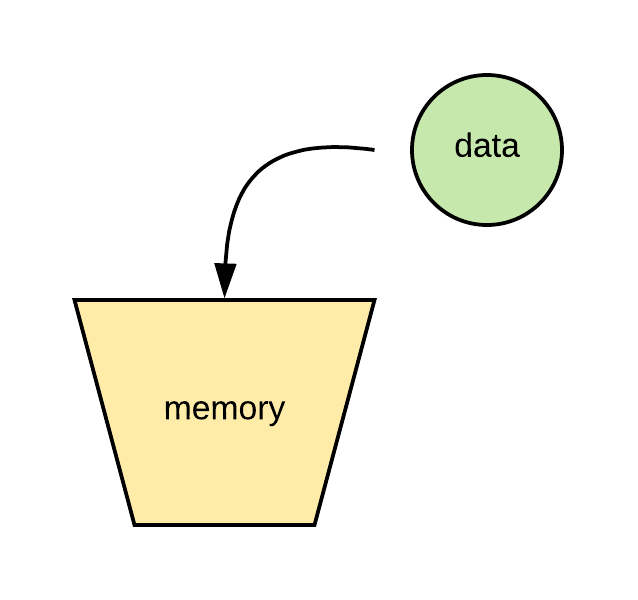Figure 1: An illustration of a memory cell holding data

In programming, a memory location is called a variable. A variable is a container for storing the data that is assigned to it. A variable is usually given a unique name by the programmer to represent a particular memory cell. The programmer can virtually call the variable any name he chooses, but it must follow a valid naming condition of only alpha-numeric lower-case characters with words separated by an underscore. Also, a variable name should have semantic meaning to the data that is stored in that variable. This helps to improve code readability later in the future.

The act of place data to a variable is called assignment.

# assigning data to a variable
> x = 1
> user_name = 'Emmanuel Okoi'


### Data Types

Python has the number and string data types in addition to other supported specialized datatypes. The number datatype, for instance, can be an int or a float. Strings are surrounded by quotes in Python.

# data types
> type(3)
'Output': int
> type(3.0)
'Output': float
> type('Jesam Ujong')
'Output': str


Other fundamental data types in Python include the lists, tuple, and dictionary. These data types hold a group of items together in sequence. Sequences in Python are indexed from 0.

Tuples are an immutable ordered sequence of items. Immutable means the data cannot be changed after being assigned. Tuple can contain elements of different types. Tuples are surrounded by brackets (...).

> my_tuple = (5, 4, 3, 2, 1, 'hello')
> type(my_tuple)
'Output': tuple
> my_tuple           # return the sixth elelment (indexed from 0)
'Output': 'hello'
> my_tuple = 'hi'    # we cannot alter an immutable data type
Traceback (most recent call last):

File "<ipython-input-49-f0e593f95bc7>", line 1, in <module>
my_tuple = 'hi'

TypeError: 'tuple' object does not support item assignment


Lists are very similar to tuples, only that they are mutable. This means that list elements can be changed after being assigned. Lists are surrounded by square-brackets [...].

> my_list = [4, 8, 16, 32, 64]
> print(my_list)    # print list items to console
'Output': [4, 8, 16, 32, 64]
> my_list        # return the fourth list elelment (indexed from 0)
'Output': 32
> my_list = 256
> print(my_list)
'Output': [4, 8, 16, 32, 256]


Dictionaries contain a mapping from keys to values. A key/value pair is an item in a dictionary. The items in a dictionary are indexed by their keys. The keys in a dictionary can be any hashable datatype (hashing transforms a string of characters into a key to speed up search). Values can be of any datatype. In other languages, a dictionary is analogous to a hash table or a map. Dictionaries are surrounded by a pair of braces {...}. A dictionary is not ordered.

> my_dict = {'name':'Rijami', 'age':42, 'height':72}
> my_dict               # dictionary items are un-ordered
'Output': {'age': 42, 'height': 72, 'name': 'Rijami'}
> my_dict['age']        # get dictionary value by indexing on keys
'Output': 42
> my_dict['age'] = 35   # change the value of a dictionary item
> my_dict['age']
'Output': 35


#### More on Lists

As earlier mentioned, because list items are mutable, they can be changed, deleted and sliced to produce a new list.

> my_list = [4, 8, 16, 32, 64]
> my_list
'Output': [4, 8, 16, 32, 64]
> my_list[1:3]      # slice the 2nd to 4th element (indexed from 0)
'Output': [8, 16]
> my_list[2:]       # slice from the 3rd element (indexed from 0)
'Output': [16, 32, 64]
> my_list[:4]       # slice till the 5th element (indexed from 0)
'Output': [4, 8, 16, 32]
> my_list[-1]       # get the last element in the list
'Output': 64
> min(my_list)      # get the minimum element in the list
'Output': 4
> max(my_list)      # get the maximum element in the list
'Output': 64
> sum(my_list)      # get the sum of elements in the list
'Output': 124
> my_list.index(16) # index(k) - return the index of the first occurrence of item k in the list
'Output': 2


When modifying a slice of elements in the list - the right-hand side can be of any length depending that the left-hand size is not a single index

# modifying a list: extended index example
> my_list[1:4] = [43, 59, 78, 21]
> my_list
'Output': [4, 43, 59, 78, 21, 64]
> my_list = [4, 8, 16, 32, 64]  # re-initialize list elementss
> my_list[1:4] = 
> my_list
'Output': [4, 43, 64]

# modifying a list: single index example
> my_list = [1, 2, 3]      # this will give a list-on-list
> my_list
'Output': [[1, 2, 3], 43, 64]
> my_list[0:1] = [1, 2, 3]    # again - this is the proper way to extend lists
> my_list
'Output': [1, 2, 3, 43, 64]


Some useful list methods include:

> my_list = [4, 8, 16, 32, 64]
> len(my_list)          # get the length of the list
'Output': 5
> my_list.insert(0,2)   # insert(i,k) - insert the element k at index i
> my_list
'Output': [2, 4, 8, 16, 32, 64]
> my_list.remove(8) # remove(k) - remove the first occurence of element k in the list
> my_list
'Output': [2, 4, 16, 32, 64]
> my_list.pop(3)    # pop(i) - return the value of the list at index i
'Output': 32
> my_list.reverse() # reverse in-place the elements in the list
> my_list
'Output': [64, 16, 4, 2]
> my_list.sort()    # sort in-place the elements in the list
> my_list
'Output': [2, 4, 16, 64]
> my_list.clear()   # clear all elements from the list
> my_list
'Output': []


The append() method adds an item (could be a list, string, or number) to the end of a list. If the item is a list, the list as a whole is appended to the end of the current list.

> my_list = [4, 8, 16, 32, 64]  # initial list
> my_list.append(2)             # append a number to the end of list
> my_list.append('wonder')      # append a string to the end of list
> my_list.append([256, 512])    # append a list to the end of list
> my_list
'Output': [4, 8, 16, 32, 64, 2, 'wonder', [256, 512]]


The extend() method extends the list by adding items from an iterable. An iterable in Python are objects that have special methods that enable you to access elements from that object sequentially. Lists and strings are iterable objects. So extend appends all the elements of the iterable to the end of the list.

> my_list = [4, 8, 16, 32, 64]
> my_list.extend(2)             # a number is not an iterable
Traceback (most recent call last):

File "<ipython-input-24-092b23c845b9>", line 1, in <module>
my_list.extend(2)

TypeError: 'int' object is not iterable

> my_list.extend('wonder')      # append a string to the end of list
> my_list.extend([256, 512])    # append a list to the end of list
> my_list
'Output': [4, 8, 16, 32, 64, 'w', 'o', 'n', 'd', 'e', 'r', 256, 512]


We can combine a list with another list by overloading the operator +

> my_list = [4, 8, 16, 32, 64]
> my_list + [256, 512]
'Output': [4, 8, 16, 32, 64, 256, 512]


#### Strings

Strings in Python are enclosed by a pair of parenthesis ''. Strings are immutable. This means they cannot be altered when assigned or when a string variable is created. Strings can be indexed like a list as well as sliced to create new lists.

> my_string = 'Schatz'
> my_string      # get first index of string
'Output': 'S'
> my_string[1:4]    # slice the string from the 2nd to the 5th element (indexed from 0)
'Output': 'cha'
> len(my_string)    # get the length of the string
'Output': 6
> my_string[-1]     # get last element of the string
'Output': 'z'


We can operate on string values with the boolean operators

> 't' in my_string
'Output': True
> 't' not in my_string
'Output': False
> 't' is my_string
'Output': False
> 't' is not my_string
'Output': True
> 't' == my_string
'Output': False
> 't' != my_string
'Output': True


We can concatenate two strings to create a new string using the overloaded operator +

> a = 'I'
> b = 'Love'
> c = 'You'
> a + b + c
'Output': 'ILoveYou'

> a + ' ' + b +  ' ' + c


### Arithmetic and Boolean Operations

#### Arithmetic Operations

In Python, we can operate on data using familiar algebra operations such as addition +, subtraction -, multiplication *, division /, and exponentiation **.

> 2 + 2     # addition
'Output': 4
> 5 - 3     # subtraction
'Output': 2
> 4 * 4     # multiplication
'Output': 16
> 10 / 2    # division
'Output': 5.0
> 2**4 / (5 + 3)    # use brackets to enforce precedence
'Output': 2.0


#### Boolean Operations

Boolean operations evaluate to True or False. Boolean operators include the comparison and logical operators. The Comparison operator includes: less than or equal to <=, less than <, greater than or equal to >=, greater than >, not equal to !=, equal to ==.

> 2 < 5
'Output': True
> 2 <= 5
'Output': True
> 2 > 5
'Output': False
> 2 >= 5
'Output': False
> 2 != 5
'Output': True
> 2 == 5
'Output': False


While the logical operators include: Boolean NOT not, Boolean AND and, Boolean OR or. We can also carry-out identity and membership tests using:

• is, is not (identity)
• in, not in (membership)
> a = [1, 2, 3]
> 2 in a
'Output': True
> 2 not in a
'Output': False
> 2 is a
'Output': False
> 2 is not a
'Output': True


### The print() statement

The print() statement is a simple way to show the output of data values to the console. Variables can be concatenated using the ,. Space is implicitly added after the comma.

> a = 'I'
> b = 'Love'
> c = 'You'
> print(a, b, c)
'Output': I Love You


#### Using the Formatter

Formatters add a placeholder for inputting a data value into a string output using the curly brace {}. The format method from the str class is invoked to receive the value as a parameter. The number of parameters in the format method should match the number of placeholders in the string representation. Other format specifiers can be added with the place-holder curly brackets.

> print("{} {} {}".format(a, b, c))
'Output': I Love You
# re-ordering the output
> print("{2} {1} {0}".format(a, b, c))
'Output': You Love I


### Control Structures

Programs need to make decisions which results in executing a particular set of instructions or a specific block of code repeatedly. With control structures, we would have the ability to write programs that can make logical decisions and execute an instruction set until a terminating condition occurs.

#### The if / elif (else-if) statements

The if / elif (else-if) statement executes a set of instructions if the tested condition evaluates to true. The else statement specifies the code that should execute if none of the previous conditions evaluate to true. It can be visualized by the flow-chart below: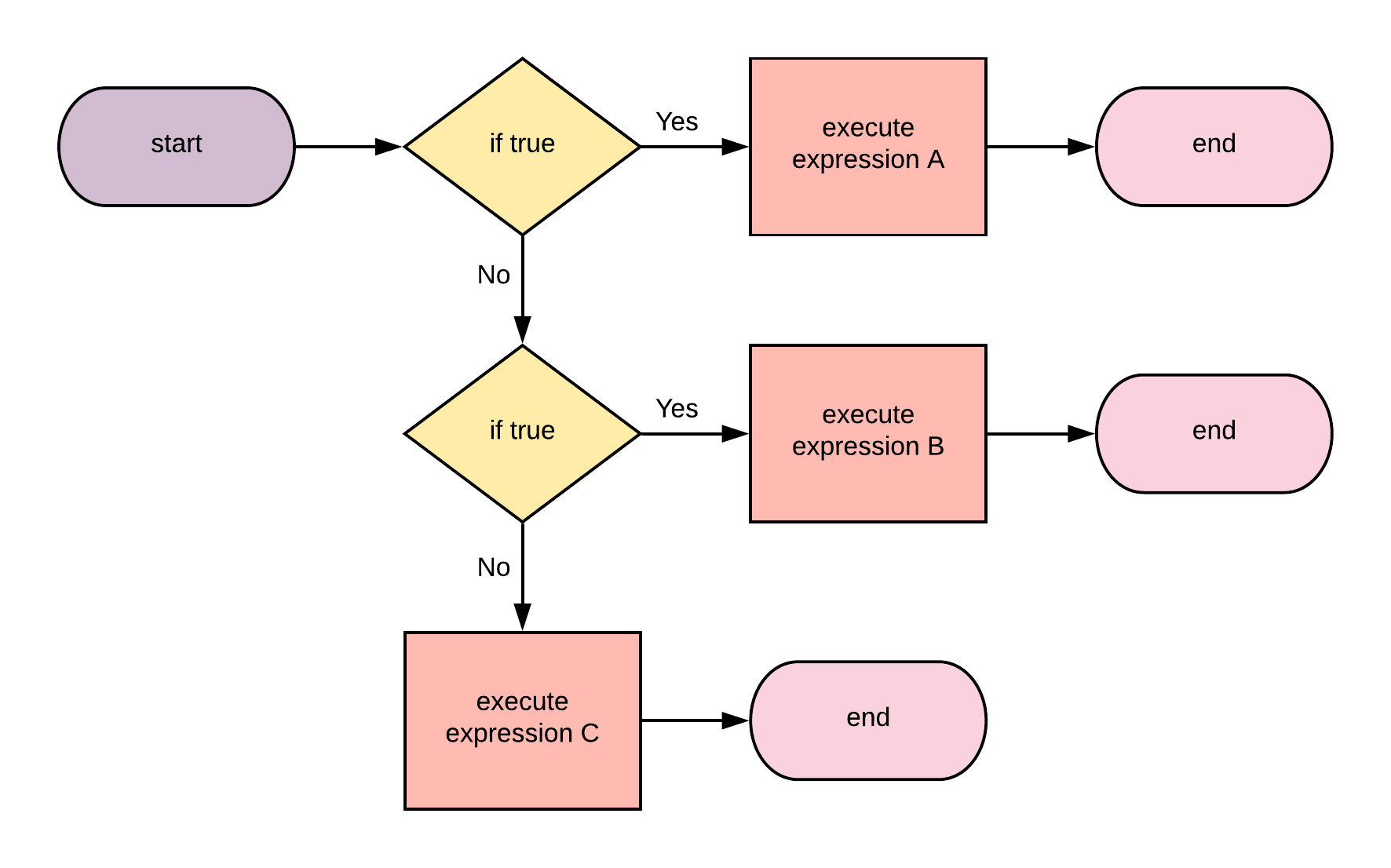Figure 2: Flowchart of the if-statement

The syntax for the if / elif statement is given as follows:

if expressionA:
statementA
elif expressionB:
statementB
...
...
else:
statementC


Here is a program example:

a = 8
if type(a) is int:
print('Number is an integer')
elif a > 0:
print('Number is positive')
else:
print('The number is negative and not an integer')

'Output': Number is an integer


#### The while loop

The while loop evaluates a condition, which if true, repeatedly executes the set of instructions within the while block. It does so until the condition evaluates to false. The while statement is visualized by the flow-chart below: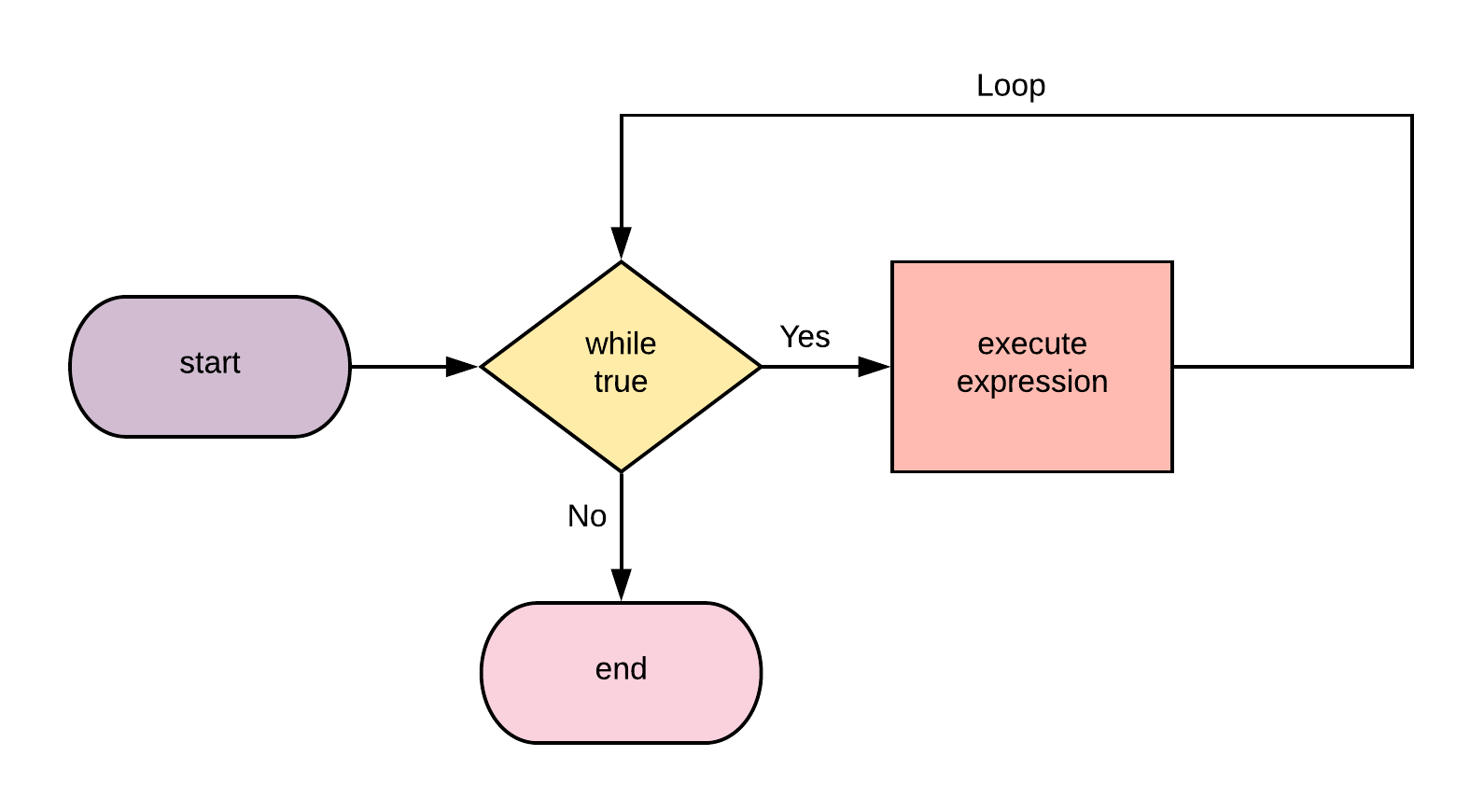Figure 3: Flowchart of the while-loop

Here is a program example:

a = 8
while a > 0:
print('Number is', a)

# decrement a
a -= 1

'Output': Number is 8
Number is 7
Number is 6
Number is 5
Number is 4
Number is 3
Number is 2
Number is 1


#### The for loop

The for loop repeats the statements within its code block until a terminating condition is reached. It is different from the while loop in that it knows exactly how many times the iteration should occur. The for loop is controlled by an iterable expression (i.e., expressions in which elements can be accessed sequentially) . The for statement is visualized by the flow-chart below: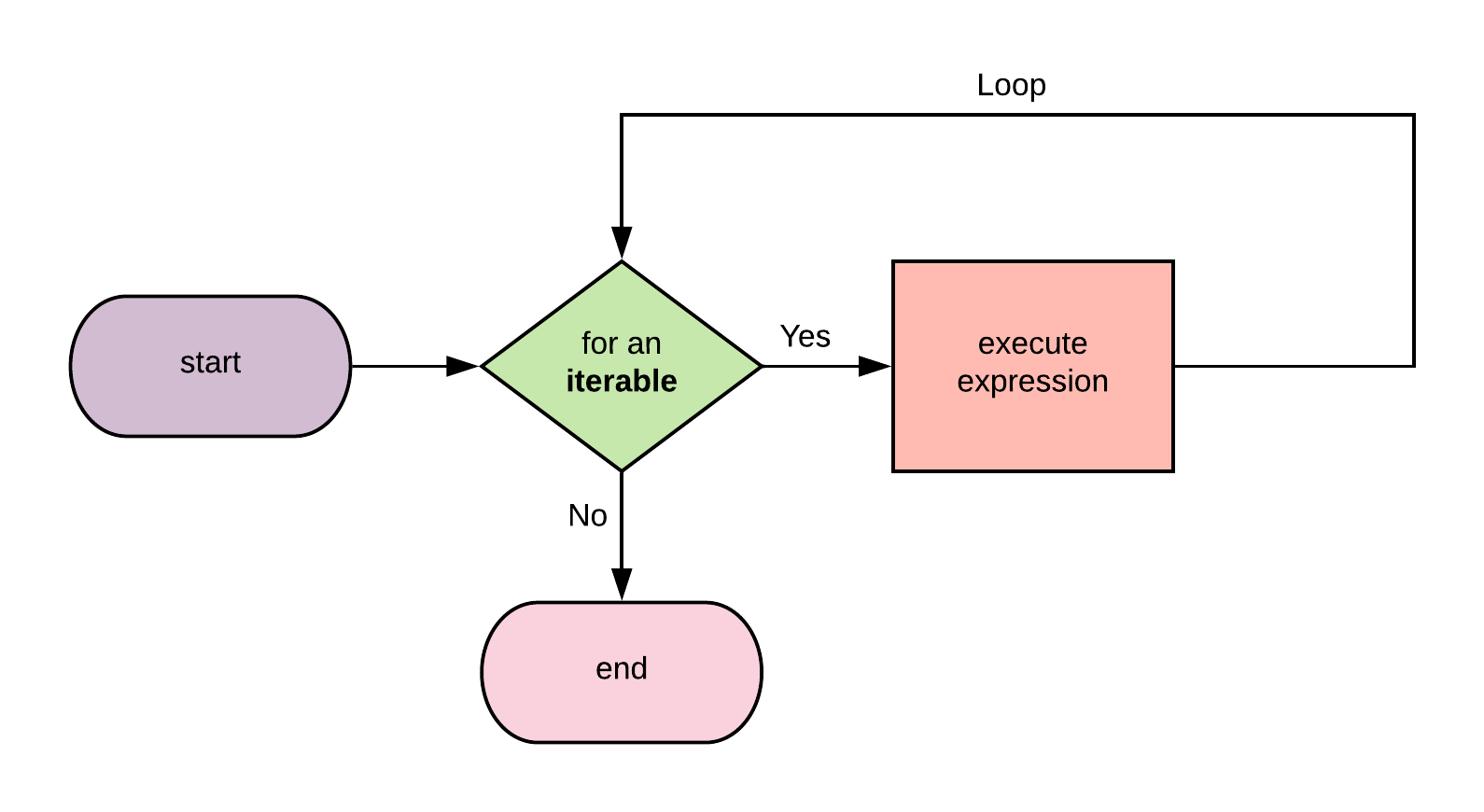Figure 4: Flowchart of the for-loop

The syntax for the for loop is as follows:

for item in iterable:
statement


Note that in in the for-loop syntax is not the same as the membership logical operator earlier discussed.

Here is a program example:

a = [2, 4, 6, 8, 10]
for elem in a:
print(elem**2)

'Output': 4
16
36
64
100


To loop for a specific number of time use the range() function.

for idx in range(5):
print('The index is', idx)

'Output': The index is 0
The index is 1
The index is 2
The index is 3
The index is 4


#### List Comprehensions

Using list comprehension, we can succinctly re-write a for-loop that iteratively builds a new list using an elegant syntax. Assuming we want to build a new list using a for-loop, we will write it as:

new_list = []
for item in iterable:
new_list.append(expression)


We can rewrite this as:

[expression for item in iterable]


Let’s have some program examples

squares = []
for elem in range(0,5):
squares.append((elem+1)**2)

> squares
'Output': [1, 4, 9, 16, 25]


The above code can be concisely written as:

> [(elem+1)**2 for elem in range(0,5)]
'Output': [1, 4, 9, 16, 25]


This is even more elegant in the presence of nested control structures

evens = []
for elem in range(0,20):
if elem % 2 == 0 and elem != 0:
evens.append(elem)

> evens
'Output': [2, 4, 6, 8, 10, 12, 14, 16, 18]


With list comprehension, we can code this as:

> [elem for elem in range(0,20) if elem % 2 == 0 and elem != 0]
'Output': [2, 4, 6, 8, 10, 12, 14, 16, 18]


#### The break and continue statements

The break statement terminates the execution of the nearest enclosing loop (for, while loops) in which it appears.

for val in range(0,10):
print("The variable val is:", val)
if val > 5:
print("Break out of for loop")
break

'Output': The variable val is: 0
The variable val is: 1
The variable val is: 2
The variable val is: 3
The variable val is: 4
The variable val is: 5
The variable val is: 6
Break out of for loop


The continue statement skips the next iteration of the loop to which it belongs; ignoring any code after it.

a = 6
while a > 0:
if a != 3:
print("The variable a is:", a)
# decrement a
a = a - 1
if a == 3:
print("Skip the iteration when a is", a)
continue

'Output': The variable a is: 6
The variable a is: 5
The variable a is: 4
Skip the iteration when a is 3
The variable a is: 2
The variable a is: 1


### Functions

A function is a code block that carries out a particular action. Functions are called by the programmer when needed by making a function call. Python comes pre-packaged with lots of useful functions to simplify programming. The programmer can also write custom functions.

A function receives data into its parameter list during a function call in which it uses to complete its execution. At the end of its execution, a function always returns a result - this result could be None or a specific data value.

Functions are treated as first-class objects in Python. That means a function can be passed as data into another function, the result of a function execution can also be a function, and a function can also be stored as a variable.

Functions are visualized as a black-box that receives a set of objects as input, executes some code and returns another set of objects as output.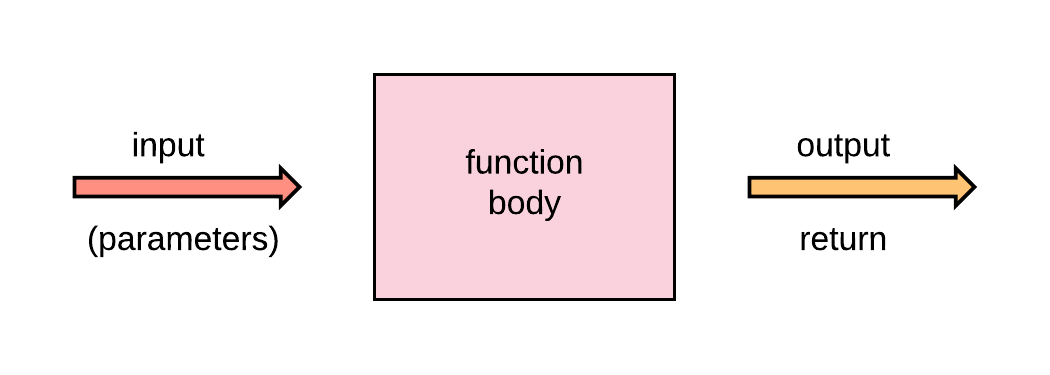Figure 5: Functions

#### User-defined functions

A function is defined using the def keyword. The syntax for creating a function is as follows:

def function-name(parameters):
statement(s)


Let’s create a simple function:

def squares(number):
return number**2

> squares(2)
'Output': 4


Here’s another function example:

def _mean_(*number):
avg = sum(number)/len(number)
return avg

> _mean_(1,2,3,4,5,6,7,8,9)
'Output': 5.0


The * before the parameter number indicates that the variable can receive any number of values - which is implicitly bound to a tuple.

#### Lambda expressions

Lambda expressions provide a concise and succinct way to write simple functions that contain just a single-line. Lambas now and again can be very useful but in general, working with def may be more readable. The syntax for lambdas are as follows:

lambda parameters: expression


Let’s see an example:

> square = lambda x: x**2
> square(2)
'Output': 4


### Packages and Modules

A module is simply a Python source-file, and packages are a collection of modules. Modules written by other programmers can be incorporated into your source-code by using import and from statements.

#### import statement

The import statement allows you to load any Python module into your source file. It has the following syntax:

import module_name [as user_defined_name][,...]


where [as user_defined_name] is optional.

Let us take an example by importing a very important package called numpy that is used for numerical processing in Python and very critical for machine learning.

import numpy as np

> np.abs(-10)   # the absolute value of -10
'Output': 10


### from statement

The from statement allows you to import a specific feature from a module into your source file. The syntax is as follows:

from module_name import module_feature [as user_defined_name][,...]


Let’s see an example:

from numpy import mean

> mean([2,4,6,8])
'Output': 5.0